4. CHEMICAL KINETICS

Q. 1. Express the relationship between degree of dissociation of an electrolyte and its molar conductivities.

Ans. ∝ =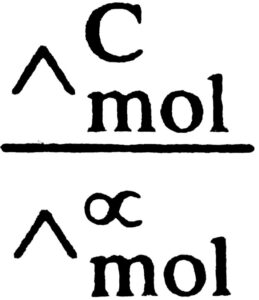Q. 2. Define the term “order of reaction for chemical reactions.

Ans. The sum of the exponents (powers) of the concentration of reactant in the rate law is termed as order of the reaction. It can be in fraction and can be zero also.

Q. 3. How does the value of rate constant vary with reactant concentration ?

Ans. For a particular reaction at a particular temperature, rate constant is constant and does not depend upon concentrations of the reactants.

Q. 4. Distinguish between ‘rate expression’ and ‘rate constant of a reaction.

Ans. Rate of reaction is defined as change in concentration of reactants or products per unit time. Its unit is mol L-1S-1.j

Q. 5. Define threshold energy of a reaction.

Ans. Threshold energy is the minimum energy which must be possessed by reacting molecules in order to undergo effective collision which leads to formation of product molecule.

Q. 6. Differentiate between Absorption and Adsorption.

Ans. Following are the main differences between Absorption and Adsorption :

 Absorption Adsorption (i) Absorption is a bulk phenomenon. (i) Addorption is a surface phenomenon. (ii) In absorption the con- centration remains same through out the material. (ii) In absorption the rate concentration on the surface is different from that in the bulk. (ii) In adsoption rate remains same throughout the process. (iii) In adsorption rate is high in the beginning and then decreases.

Q. 7. Explain Kohlrausch’s law of indepent migration of ions. Mention one application of Kohlrausch’s law.

Ans. It states that at infinite dilution, molar conductivity of an electrolyte is equal to sum of contribution due to cation as well as anion e.g.,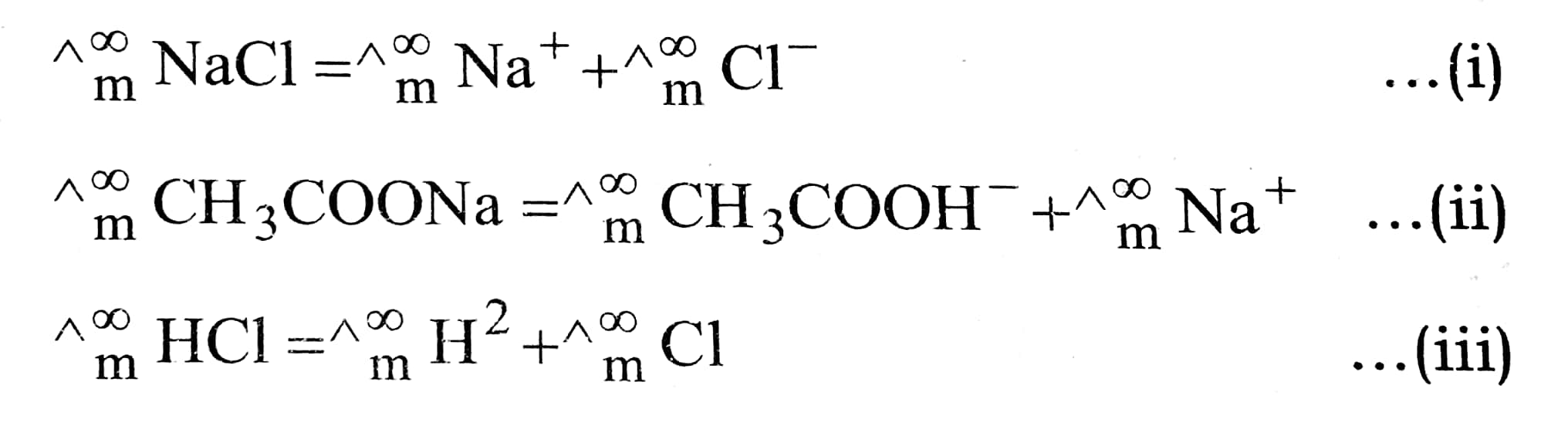It helps to determine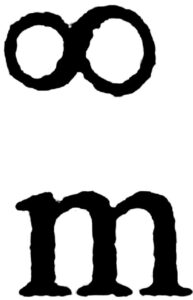CH3COOH by adding equation (ii) and (iii) and substracting (i).

Q. 8. What is a salt bridge ? What is its significance ?

Ans. Salt bridge : A salt bridge consists of a saturated solution of NH4NO3 or KCl mixed with gelatin or agar jelly filled in a glass tube bent according to the requirement of the experiment.

Significance :

(i) Salt bridge prevents mixing of two electrolytes.
(ii) Prevents junction potential.
(iii) Maintains electrical neurality.

Q. 9. What is the initial rate method for the determination of a reaction rate when more than one reactant is present ?

Ans. Rl = dx/dt = k[Al]a[Bl]b

R2 = k[A2]a[Bl]b

Dividing (ii) by (i), we get

.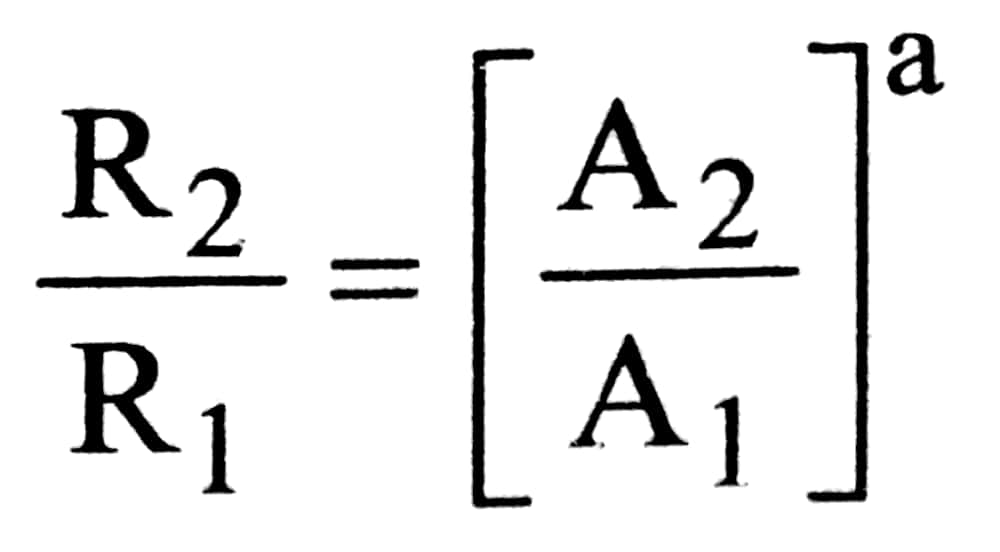From this can be calculate

R3/R1 = k[A1]a[B2]b

(iii) Dividing (iii) by (i), we get

.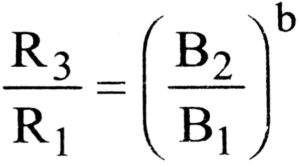From this we can calculate b.

Q. 10. The rate Law for the decomposition of N2O5is

Rate = k [N2O5]

What is the significace of k in this equation ?

Ans. If [N2O5] = 1; then Rate = k.

i.e., k is equal to the rate of the rection when concentration of N2O5 is unity, i.e., 1 mol L-1.

Q. 11. Write expression for half-life in case of a rections between hydrogen and chlorine to from hydrochloric gas.

Ans. Given reaction is zero order reaction. Hence t1/2 = [A]0/2k.

Q. 12. For the assumed reaction X2 + 3Y2 → 2XY3, write the rate equation in terms of rate of disapperarance of Y2.

Ans. Rate =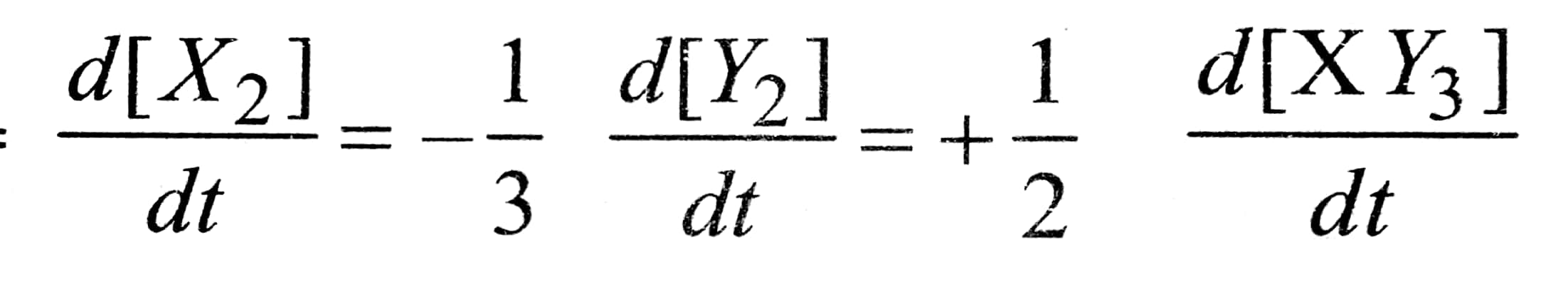Rate of disappearance of y2 =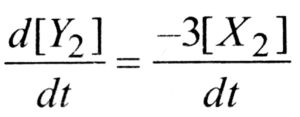=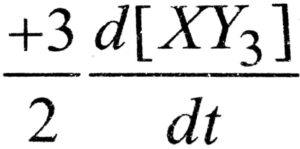Q. 13. The reaction A + B → C has zero order. What is the rate equation ?

Ans. Rate = k [A]° [B]° = k.

Q. 14. Express the relation between half life period of a reactant with initial concentration for a reaction of nth order.

Ans. t1/2α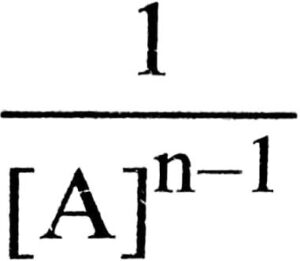Q. 15. How does the value of rate constant vary with reactant concentration ?

Ans. For reaction of nth ordr =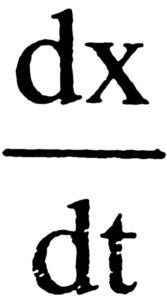= Rate = k[ conc.]n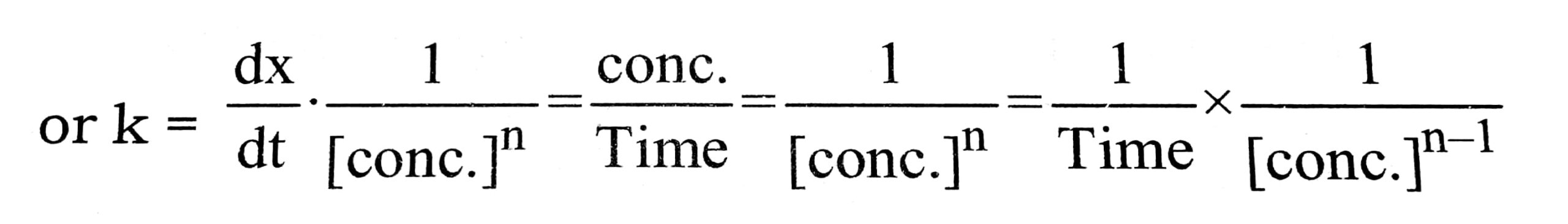i.e.,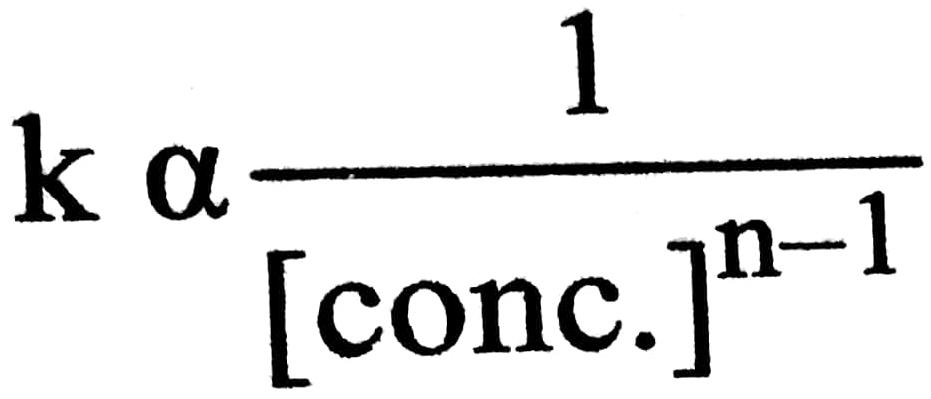Q. 16. If half-life period of a reaction is inversely proportional to initial concentration of a rectant, what is the order of the reaction ?

Ans. Two, this is because for 2nd order reactions t1/2 1/ ka.

Q. 17. Give one example of a first order reaction.

Ans. N2 O5 → N2 O4 +1/2 O2

Q. 18. For a reaction A + B, the rate of the reaction can be denoted by – dA/ dt or + dB/ dt. State the significance of plus and minus signs in this case.

Ans. Minus sign indicates the decrease in the concentration of the reactant while plus sign indicate an increase in the conc. of the product with time.

Q. 19. Why rate of reaction does not remain constant throughout ?

Ans. Because it depends upon concertration of the reactions which keeps on decreasing with time.

Q. 20. What is the relationship between rate constant and activation energy of a reactions ?

Ans. K = A e-Ea/RT is Arrhenius equation.

Q. 21. Why hydrolysis of ethyl acetate with NaOH is a second order reaction while with HCl it is a Ist order reaction ?

Ans. Rate of hydrolysis of ethyl acetate with NaOH depends upon conc. of both while that with HCI, it depends upon only the conc. of ethyl acetate.

Q. 22. Define specific reaction rate or rate constant.

Ans. It is the rate of a reaction when the molar concentration of each of the reactant is unity.

Q. 23. What is the rate determine step of a reactions ?

Ans. The slowest step of a multi-step reaction is called its rate determining step.

Q. 24. Give an example of a reaction having fractional order.

Ans. Decomposition of acetaldehyde (order is 1.5)

CH3 CHOCH4 + CO

Q. 25. A substance with initial concentration a follows zero order kinetics. In how much time will the reaction go to completion ?

Ans.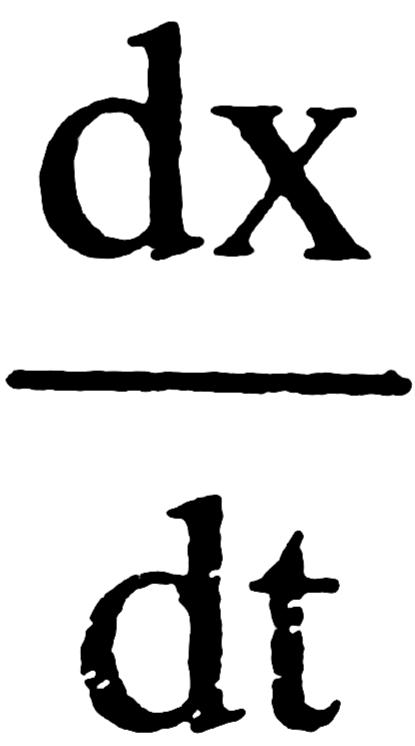= k or dx = k dt ∴ x = kt + I

when t = 0; x = 0 ∴ I = 0.

Hence x = kt or t = x/K, For completion x = a ∴ t = a / K.

Q. 26. In some cases, it is found that a large no. of colliding molecules have energy more than threshold energy, yet the reaction is slow, why ?

Ans. The colliding molecules may not have proper orientation at the time of collision.

Q. 27. Give an example of a pseudo first order reaction.

Ans. Acid catalysed hydrolysis of ethyl acetate.

CH3 COOC3H5 + H2O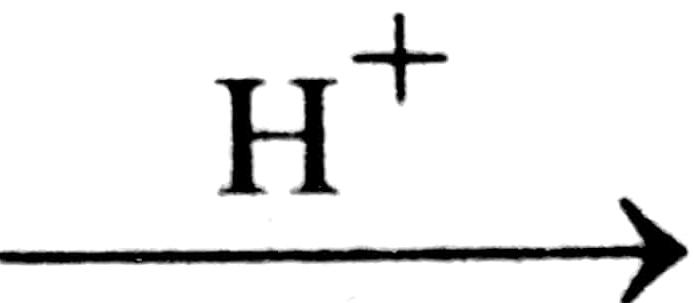H3COOH + C2H5OH
Rate = [CH3COOC2H5)

Q. 28. What is meant by an elementary reaction ?

Ans. A reaction which takes place in one step P is called an clementary reaction.

Q. 29. How is half-life period related to initial concentration of a second order reaction ?

Ans. t1 / 2 α a1-n.For n = 2, t1 / 2 ∝ a-1, i.e. t1 / 2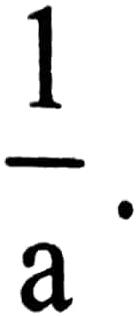Thus half life period of a second order reaction is inversely proportional to the inital concentration of the reactant.

Q. 30. Give one example of a reaction where order and molecularity are equal.

Ans. Order and molecularity are same in a single step reaction.

eg. 2HI (g) → H2 (g) + I2 (g)

Q. 31. State any one condition under which a bimolecular reaction may be kinetically of first order.

Ans. A bimolecular reaction may become kinetically of the first order is one of the reactants is present in excess.

Q. 32. Rate of a reaction is given by the equation : Rate = k [A]2 [B]. What are the units for the rate and rate constant for this reaction ?

Ans. Units of rate = mol L-1 s-1.

Units of k =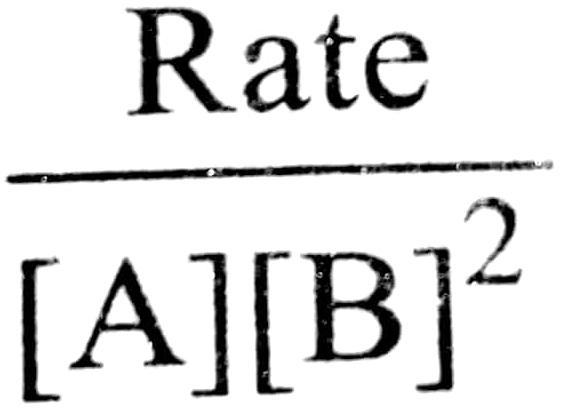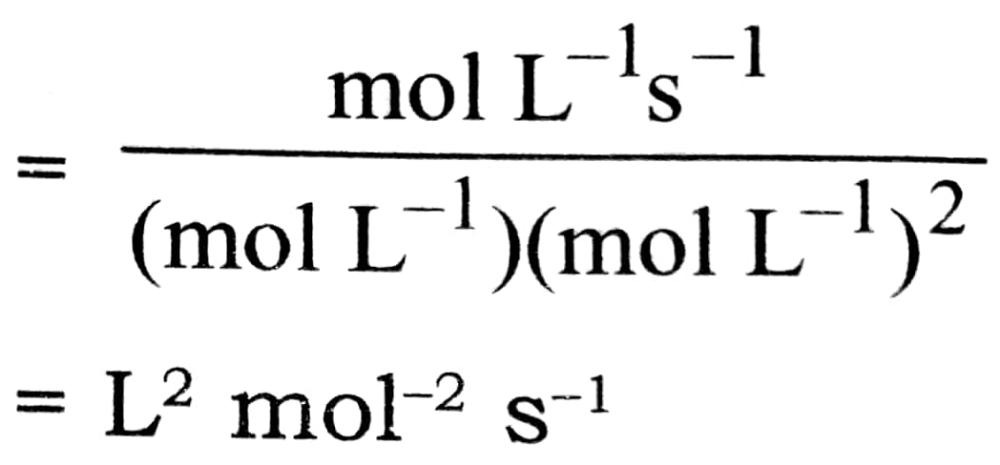Q. 33. How is the rate constant of a reaction related to the concentration of the reactants ?

Ans. Rate constant does not depend upon the concentration of the reactants.

Q. 34. The rate law for the reaction : Ester + H+ → Acid+ alcohol is dx/dt = k [Ester] [H+]॰. What would be the effect on the rate if (i) concentration of the ester is doubled, (ii) concentration of H+ is doubled ?

Ans. (i) The rate of the reaction will be doubled. (ii) No effect on rate.

Q. 35. The form of the rate law for a reaction is expressed as : rate = k [C12] [NO]2. Find out the orders of the reaction with respect to C12 and with respect to NO and also the overall order of the reaction.

Ans. Order w.r.t. Cl2 = 1; Order w.r.t. NO = 2
Over-all order = 1 + 2 = 3

## Class 12th Chemistry Short Type Question English

 S.N CHEMISTRY SHORT TYPE QUESTION 2022 1 SOLID STATE 2 SOLUTION 3 ELECTROCHEMISTRY 4 CHEMICAL KINETICS 5 SURFACE CHEMISTRY 6 GENERAL PRINCIPLES AND PROCESSES OF ISOLATION OF ELEMENTS 7 THE P-BLOCK ELEMENTS 8 THE D- AND f-BLOCK ELEMENTS 9 CO-ORDINATION COMPOUNDS 10 HALOALKANES AND HALOARENES 11 ALCOHOLS, PHENOLS AND ETHERS 12 ALDEHYDES, KETONES AND CARBOXYLIC ACIDS 13 AMINES 14 BIOMOLECULES 15 POLYMERS 16 CHEMISTRY IN EVERYDAY LIFE